Documentation

# subplot

Create axes in tiled positions

## Syntax

``subplot(m,n,p)``
``subplot(m,n,p,'replace')``
``subplot(m,n,p,'align') ``
``subplot(m,n,p,ax)``
``subplot('Position',pos)``
``subplot(___,Name,Value)``
``ax = subplot(___)``
``subplot(ax)``

## Description

example

````subplot(m,n,p)` divides the current figure into an `m`-by-`n` grid and creates axes in the position specified by `p`. MATLAB® numbers subplot positions by row. The first subplot is the first column of the first row, the second subplot is the second column of the first row, and so on. If axes exist in the specified position, then this command makes the axes the current axes.```

example

````subplot(m,n,p,'replace')` deletes existing axes in position `p` and creates new axes.```
````subplot(m,n,p,'align') ` creates new axes so that the plot boxes are aligned. This option is the default behavior.```

example

````subplot(m,n,p,ax)` converts the existing axes, `ax`, into a subplot in the same figure.```

example

````subplot('Position',pos)` creates axes in the custom position specified by `pos`. Use this option to position a subplot that does not align with grid positions. Specify `pos` as a four-element vector of the form ```[left bottom width height]```. If the new axes overlap existing axes, then the new axes replace the existing axes.```
````subplot(___,Name,Value)` modifies axes properties using one or more name-value pair arguments. Set axes properties after all other input arguments.```

example

````ax = subplot(___)` creates an `Axes` object, `PolarAxes` object, or `GeographicAxes` object. Use `ax` to make future modifications to the axes.```

example

````subplot(ax)` makes the axes specified by `ax` the current axes for the parent figure. This option does not make the parent figure the current figure if it is not already the current figure.```

## Examples

collapse all

Create a figure with two stacked subplots. Plot a sine wave in each one.

```subplot(2,1,1); x = linspace(0,10); y1 = sin(x); plot(x,y1) subplot(2,1,2); y2 = sin(5*x); plot(x,y2)```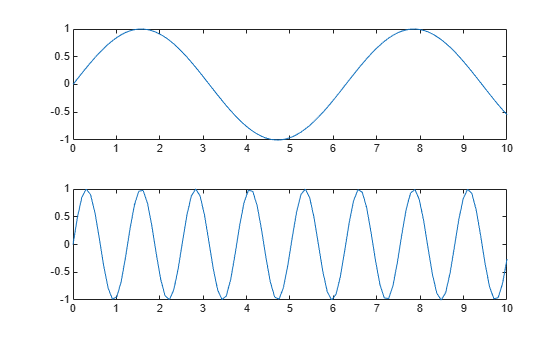Create a figure divided into four subplots. Plot a sine wave in each one and title each subplot.

```subplot(2,2,1) x = linspace(0,10); y1 = sin(x); plot(x,y1) title('Subplot 1: sin(x)') subplot(2,2,2) y2 = sin(2*x); plot(x,y2) title('Subplot 2: sin(2x)') subplot(2,2,3) y3 = sin(4*x); plot(x,y3) title('Subplot 3: sin(4x)') subplot(2,2,4) y4 = sin(8*x); plot(x,y4) title('Subplot 4: sin(8x)')```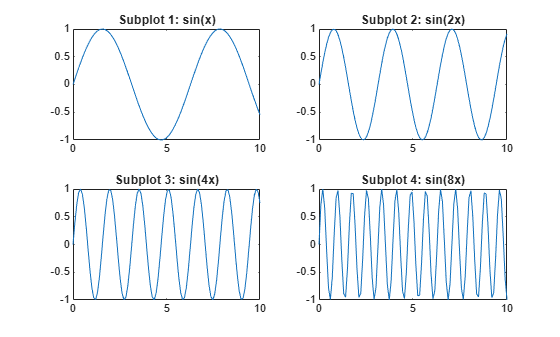Create a figure containing with three subplots. Create two subplots across the upper half of the figure and a third subplot that spans the lower half of the figure. Add titles to each subplot.

```subplot(2,2,1); x = linspace(-3.8,3.8); y_cos = cos(x); plot(x,y_cos); title('Subplot 1: Cosine') subplot(2,2,2); y_poly = 1 - x.^2./2 + x.^4./24; plot(x,y_poly,'g'); title('Subplot 2: Polynomial') subplot(2,2,[3,4]); plot(x,y_cos,'b',x,y_poly,'g'); title('Subplot 3 and 4: Both')```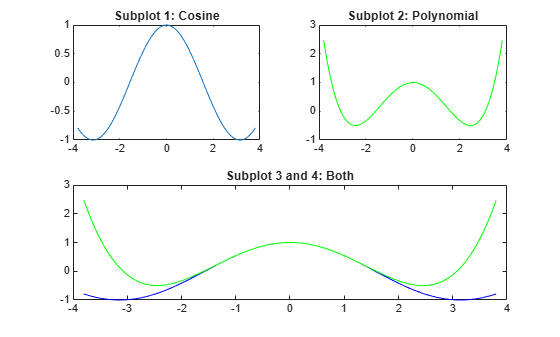Create a figure with four stem plots of random data. Then replace the second subplot with empty axes.

```for k = 1:4 data = rand(1,10); subplot(2,2,k) stem(data) end```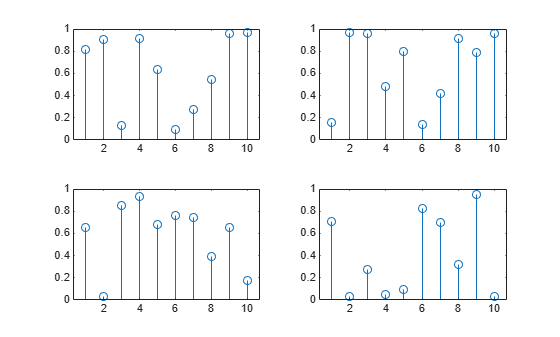`subplot(2,2,2,'replace')`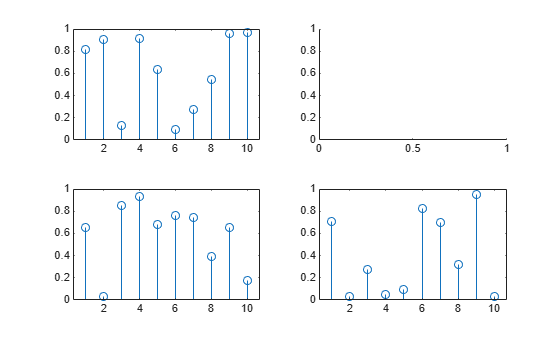Create a figure with two subplots that are not aligned with grid positions. Specify a custom position for each subplot.

```pos1 = [0.1 0.3 0.3 0.3]; subplot('Position',pos1) y = magic(4); plot(y) title('First Subplot') pos2 = [0.5 0.15 0.4 0.7]; subplot('Position',pos2) bar(y) title('Second Subplot')```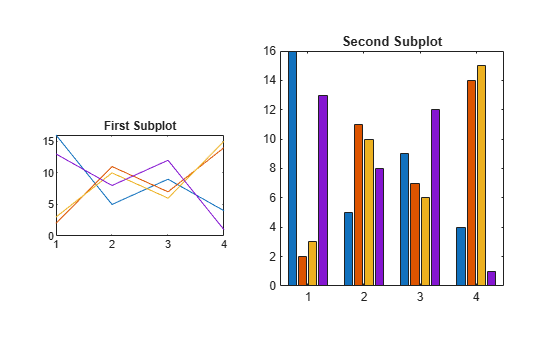Create a figure with two polar axes. Create a polar line chart in the upper subplot and a polar scatter chart in the lower subplot.

```figure ax1 = subplot(2,1,1,polaraxes); theta = linspace(0,2*pi,50); rho = sin(theta).*cos(theta); polarplot(ax1,theta,rho) ax2 = subplot(2,1,2,polaraxes); polarscatter(ax2,theta,rho)```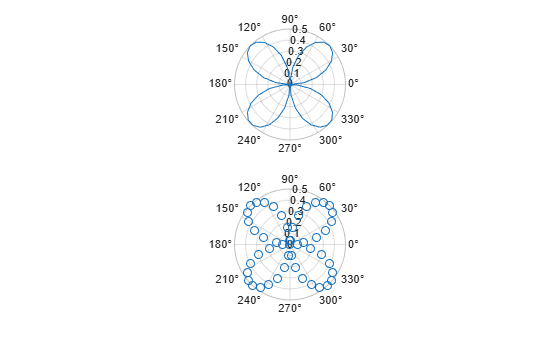Create a figure with two subplots. Assign the `Axes` objects to the variables `ax1` and `ax2`. Specify the `Axes` objects as inputs to the plotting functions to ensure that the functions plot into a specific subplot.

```ax1 = subplot(2,1,1); Z = peaks; plot(ax1,Z(1:20,:)) ax2 = subplot(2,1,2); plot(ax2,Z)```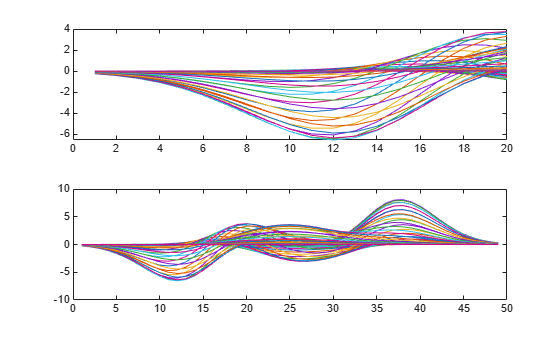Modify the axes by setting properties of the `Axes` objects. Change the font size for the upper subplot and the line width for the lower subplot. Some plotting functions set axes properties. Execute plotting functions before specifying axes properties to avoid overriding existing axes property settings.

Note: Starting in R2014b, you can use dot notation to set properties. If you are using an earlier release, use the `set` function instead.

```ax1.FontSize = 15; ax2.LineWidth = 2;```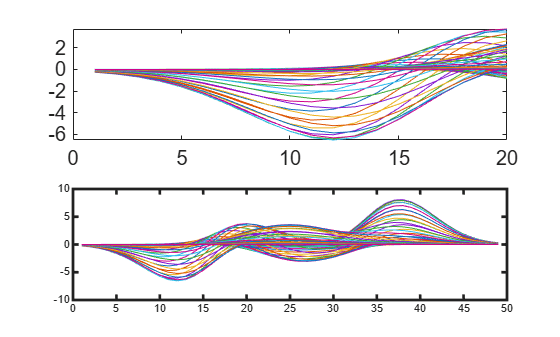Create a figure with multiple subplots. Store the `Axes` objects in vector `ax`. Then make the second subplot the current axes. Create a line chart and change the axis limits for the second subplot. By default, graphics functions target the current axes.

```for k = 1:4 ax(k) = subplot(2,2,k); end subplot(ax(2)) x = linspace(1,50); y = sin(x); plot(x,y,'Color',[0.1, 0.5, 0.1]) title('Second Subplot') axis([0 50 -1 1])```Create a line chart. Then convert the axes so that it is the lower subplot of the figure. The subplot function uses the figure in which the original axes existed.

```x = linspace(1,10); y = sin(x); plot(x,y) title('Sine Plot')```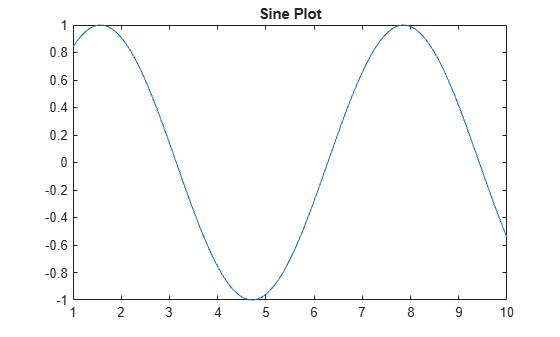```ax = gca; subplot(2,1,2,ax)```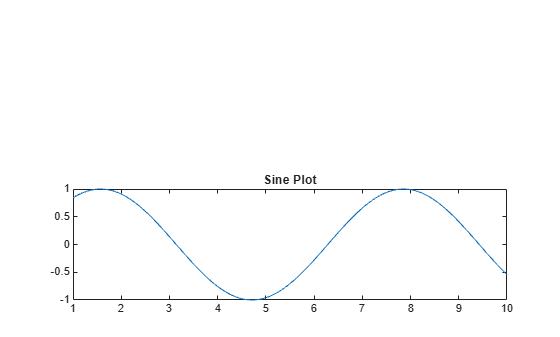Combine axes that exist in separate figures in a single figure with subplots.

Create two plots in two different figures. Assign the `Axes` objects to the variables `ax1` and `ax2`. Assign the `Legend` object to the variable `lgd`.

```figure x = linspace(0,10); y1 = sin(x); plot(x,y1) title('Line Plot 1')``````ax1 = gca; figure y2 = 2*sin(x); plot(x,y2) title('Line Plot 2') lgd = legend('2*Sin(x)');````ax2 = gca;`

Create copies of the two `Axes` objects using `copyobj`. Specify the parents of the copied axes as a new figure. Since legends and colorbars do not get copied with the associated axes, copy the legend with the axes.

```fnew = figure; ax1_copy = copyobj(ax1,fnew); subplot(2,1,1,ax1_copy) copies = copyobj([ax2,lgd],fnew); ax2_copy = copies(1); subplot(2,1,2,ax2_copy)```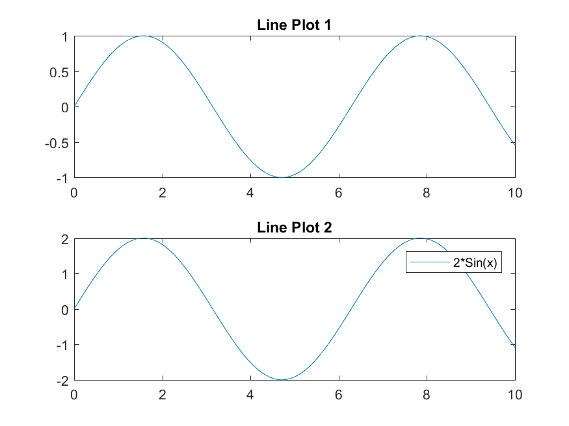## Input Arguments

collapse all

Number of grid rows, specified as a positive integer.

Data Types: `single` | `double`

Number of grid columns, specified as a positive integer.

Data Types: `single` | `double`

Grid position for the new axes, specified as a scalar or vector of positive integers.

• If `p` is a scalar positive integer, then `subplot` creates a subplot in grid position `p`.

• If `p` is a vector of positive integers, then `subplot` creates a subplot that spans the grid positions listed in `p`.

Example: `subplot(2,3,1)` creates a subplot in position 1.

Example: `subplot(2,3,[2,5])` creates a subplot spanning positions 2 and 5.

Example: `subplot(2,3,[2,6])` creates a subplot spanning positions 2, 3, 5, and 6.

Data Types: `single` | `double`

Custom position for the new axes, specified as a four-element vector of the form `[left bottom width height]`.

• The `left` and `bottom` elements specify the position of the bottom-left corner of the subplot in relation to the bottom-left corner of the figure.

• The `width` and `height` elements specify the subplot dimensions.

Specify values between `0` and `1` that are normalized with respect to the interior of the figure.

### Note

When using a script to create subplots, MATLAB does not finalize the `Position` property value until either a `drawnow` command is issued or MATLAB returns to await a user command. The `Position` property value for a subplot is subject to change until the script either refreshes the plot or exits.

Example: `subplot('Position',[0.1 0.1 0.45 0.45])`

Data Types: `single` | `double`

Existing axes to make current or convert to a subplot, specified as an `Axes` object, a `PolarAxes` object, a `GeographicAxes` object, or a graphics object with an `ActivePositionProperty` property, such as a `HeatmapChart` object.

To create empty polar or geographic axes in a subplot position, specify `ax` as the `polaraxes` or `geoaxes` function. For example, `subplot(2,1,2,polaraxes)`.

### Name-Value Pair Arguments

Specify optional comma-separated pairs of `Name,Value` arguments. `Name` is the argument name and `Value` is the corresponding value. `Name` must appear inside quotes. You can specify several name and value pair arguments in any order as `Name1,Value1,...,NameN,ValueN`.

Example: `subplot(m,n,p,'XGrid','on')`

Some plotting functions override property settings. Consider setting axes properties after plotting. The properties you can set depend on the type of axes:

.

## Tips

• To clear the contents of the figure, use `clf`. For example, you might clear the existing subplot layout from the figure before creating a new subplot layout.

• To overlay axes, use the `axes` command instead. The `subplot` function deletes existing axes that overlap new axes. For example, ```subplot('Position',[.35 .35 .3 .3])``` deletes any underlying axes, but ```axes('Position',[.35 .35 .3 .3])``` positions new axes in the middle of the figure without deleting underlying axes.

• `subplot(111)` is an exception and not identical in behavior to `subplot(1,1,1)`. For reasons of backwards compatibility, `subplot(111)` is a special case of subplot that does not immediately create axes, but sets up the figure so that the next graphics command executes ```clf reset```. The next graphics command deletes all the figure children and creates new axes in the default position. `subplot(111)` does not return an `Axes` object and an error occurs if code specifies a return argument.

## Alternative Functionality

Use the `tiledlayout` and `nexttile` functions to create a configurable tiling of plots. The configuration options include:

• Control over the spacing between the plots and around the edges of the layout

• An option for a shared title at the top of the layout

• Options for shared x- and y-axis labels

• An option to control whether the tiling has a fixed size or variable size that can reflow